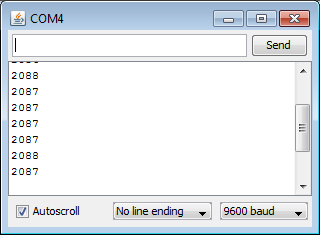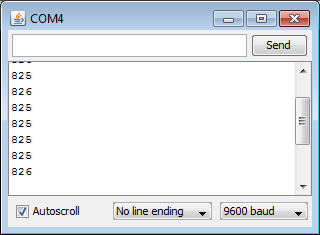## How Fast is the Arduino PID Library?

A couple people have asked me about speed since v1 was released. Since I had no idea, I decided I should do a little benchmarking. (Tests were done on a Duemilanove with the ATMega168.)

### The Test

```#include <PID_v1.h>

double Setpoint, Input, Output;
PID myPID(&Input, &Output, &Setpoint,2,5,1, DIRECT);

void setup()
{
Setpoint = 100;

myPID.SetMode(AUTOMATIC);
Serial.begin(9600);
}

void loop()
{
unsigned long startTime = millis();
/*Do a PID calculation 10000 times*/
for(int i=0;i<10000;i++)
{
myPID.Compute();
analogWrite(3,Output);
}
/*print the elapsed time*/
Serial.println(millis()-startTime);
}
```

The code above is a modification of the Basic PID example. Rather than do the pid stuff once though, it calls it 10000 times in a row. Dividing the resulting elapsed time by 10000 gives us precision down into the microsecond range.

Not pictured in this code, I also went into PID_v1.cpp and made SampleTime=0. That ensured that every time Compute() was called, the PID equation was evaluated.

One last note. Notice that analogRead and analogWrite are in the for loop. I couldn’t think of a situation where you’d have a PID without also having those functions somewhere in the loop, so I included them in the test.

### The Results2088 mSec / 10000 = 0.21 mSec
Ok. Pretty fast. Now just for fun, I commented out the read and write code (lines 21 & 23) to see how much of the 0.21 was due to the Compute function:826mSec / 10000 = 0.08 mSec
So the PID was only responsible for about a third of the total time. Unless I’m missing something (please tell me if I am,) it looks like the Compute function is only slightly slower than an analog read or an analog write!

### Some Closing Thoughts

Looking at these results, your first inclination might be to make the sample time as small as possible (1 mSec.) After all, that’s WAY bigger than the 0.08 mSec required. 2 things to remember:

1. There’s other code in your program. All together it may add up to more than 1mSec
2. Even if your loop time is less than 1 mSec (say it’s 0.7 mSec,) Compute only gets called once per loop, so the pid will actually be evaluated every 1.4 mSec.

Bottom line, if you’re looking to evaluate the pid really quickly, you need to be careful to avoid weird performance.

Tags: ,

### 2 Responses to “How Fast is the Arduino PID Library?”

1.Rinson Antony T.M says:

So it means that .. the maximum PID compute speed will be obtained when we set the sampletimpe equal to zero. Right ?

2.Brett says:

Sort of. Remember that the way the library is written, sample time is part of the tuning parameters. That code would need to be modified. Honestly, if you were going to make the sample time 0, it would probably make more sense just to remove all the code that was added in the sample time modification. If you did that though, you’d need to find a way to ensure that Compute was called at a regular interval (maybe the MSTimer2 or Timer1 libraries?)